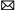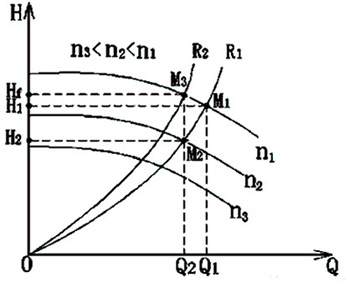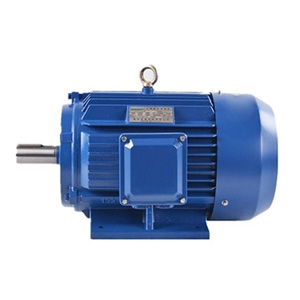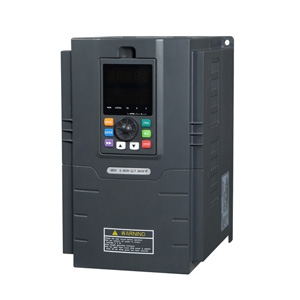Home » FAQ » How Does VFD Accelerate or Decelerate Motor?

# How Does VFD Accelerate or Decelerate Motor?

The motor acceleration dω/dt depends on the acceleration torque, but the frequency change rate of VFD in starting and braking process is set according to the requirements of frequency conversion speed control system.

Changing frequency (hertz) can change the motor speed. For example, the speed of a four-pole motor is 1500 rpm at the frequency of 50Hz, but it changes to 1800 rpm when the frequency is changed to 60 Hz, so the lower the frequency, the lower the speed.

Relation: n=f（1-s）60／p

1. Asynchronous motor, stator speed and magnetic field speed N1＝60f/p
2. Rotor speed and synchronized speed asynchronism, slippage doesn’t have an accurate formula due to the changes of load.
3. Only rated slippage can be calculated, then rotor speed is estimated according to the rated slippage and reference load of synchronized slippage.
4. That means there’s no accurate calculation formula for the rotor speed, which is impossible.

n=60f/p refers to the synchronization speed. Actually, it's not that complicated. Based on the motor speed marked on the nameplate, if the speed is 50Hz, the marked motor speed is 1450rpm. When the frequency is 25Hz, the speed becomes 725rpm. When the frequency is 5Hz, the speed becomes 145rpm.

The motor acceleration dω/dt depends on the acceleration torque, but the frequency change rate of VFD in starting and braking process is set according to the requirements of frequency conversion speed control system. If the motor’s rotational inertia and the change rate of motor load are accelerated or decelerated according to the predetermined speed, insufficient acceleration torque may lead to motor stall, namely the motor speed is inconsistent with the output frequency of the variable frequency drive, thus resulting in the over-current or over-voltage of VFD.

Therefore, it’s necessary to establish the acceleration and deceleration time according to the motor’s inertia and actual load, so that the frequency change rate of converter can be coordinated with the speed change rate of the motor. Check whether the setting is reasonable. If the VFD overcurrent occurs in the starting process, the acceleration time can be prolonged appropriately. If the VFD overcurrent occurs in the braking process, the deceleration time can be prolonged appropriately. In other hand, both acceleration and deceleration time should not be set too long, too long time will affect the production efficiency, especially when frequency control system is frequently started and braked.

Plz Calculate (7 - 2) =
(Your comment will show after approved.)

You may also like:Variable Frequency Drivesinfo@variablefrequencydrive.net

Featured Articles
Variable Frequency Drive for FansFan is a kind of machine that compresses and conveys gas. If the pressure of exhausted air is quite low, the fan plays a role of ...
Variable Frequency Drive for Food ...Nowadays the food machinery industry has been rapidly developed. As the motor drive, variable frequency drive will change the ...
Can I Run a 60Hz Motor on 50Hz by ...We are often asked “can the motor with a rated frequency of 60Hz operate at 50Hz (vice versa)?” When the motor is operated at a ...
How Does Variable Frequency Drive ... The rotating speed of inductor type AC motor (hereinafter referred to as motor) is determined by the number of pole and ...
What is a Variable Frequency DriveVariable frequency drive (VFD) is an adjustable speed drive used for motor drive system, control the speed and torque of the AC ...